# Free Electron Theory of Metals

The metals form a unique type of bonding known as metallic bonding and form the lattice structure. The uniqueness in such a type of bonding fashion lies in the fact that unlike ionic bonding and covalent bonding where the sharing of electrons is between two atoms and the electrons remain localized, in metallic bonding the bond is formed among all the atoms in the lattice and the free electrons from each atom is shared by the whole lattice. These free electrons move freely throughout the lattice and hence are termed as electron gas.
Neglecting the electron-electron interaction and the electron-ion interaction, it appears as if the electrons move in a confined box with periodic collision with ions in the lattice. This idea was given by Drude and he utilized it to explain many properties of metals satisfactorily such as electrical conductivity, thermal conductivity etc.

Drude applied equations of simple mechanics on the electrons to derive several expressions and also arrive at the Ohm’s Law. Normally the electrons are in a random motion throughout the lattice, which is primarily due to thermal energy, and the net average effect turns out to be zero. However when electric field is applied to the metal, another component of velocity is superimposed on each electron due to the force acting on it by virtue of its charge.

According to Newtonian mechanics we can write-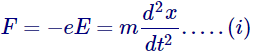Where, e= charge on electron,
E = applied electric field in V/m
m = mass of electron
x = distance in the direction of motion.

Integrating equation (i)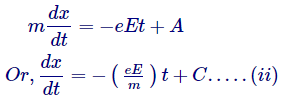Where, A and C are constants.

Equation (ii) is the equation of velocity of electrons, hence C has dimension of velocity, and can only be random velocity of electron which it had at the initial stage when no field was applied. Hence,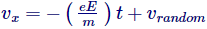However, as we discussed earlier this random velocity averages out to zero, thus the average velocity of the electrons can be written as-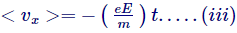The above equation indicates that the velocity keeps on increasing indefinitely with time until E is switched on, however this is not possible. The explanation to this is given by saying that the electrons do not move freely in the lattice, rather they collide with the ions present in the lattice structure, and loose their velocity and again gets accelerated and again collides and so on.

Hence seeing the average effect we consider that on an average the time between two collisions is T, known as the relaxation time or collision time and the average velocity attained by electrons in T time period is known as drift velocity.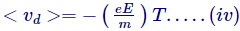Now, for number of electrons per unit volume as n, the amount of charge passing through a cross section A in time dt will be given by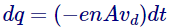Hence the current flowing will be given by,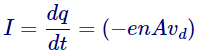And hence the current density will be,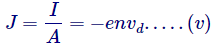Putting the value of drift velocity from equations (iv) in (v),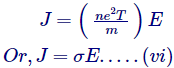Which is nothing but the Ohm’s Law itself, where,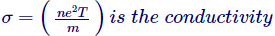Now here we define a new term known as mobility, defined as drift velocity per unit electric field,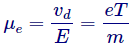Its unit is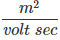We also observe from the expression of conductivity that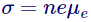Want To Learn Faster? 🎓
Get electrical articles delivered to your inbox every week.
No credit card required—it’s 100% free.